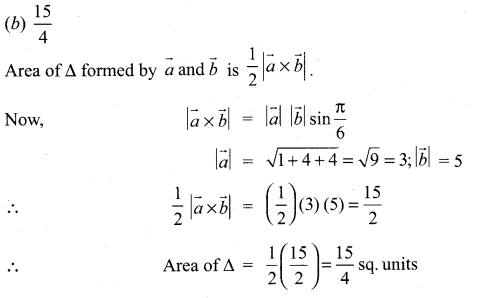# Samacheer Kalvi 11th Maths Solutions Chapter 8 Vector Algebra – I Ex 8.5

## Tamilnadu Samacheer Kalvi 11th Maths Solutions Chapter 8 Vector Algebra – I Ex 8.5

Choose the correct or the most suitable answer from the given four alternatives:

Question 1.
The value of $$\overrightarrow{\mathrm{AB}}+\overrightarrow{\mathrm{BC}}+\overrightarrow{\mathrm{DA}}+\overrightarrow{\mathrm{CD}}$$ is ………………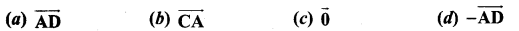Solution:
(c) $$\overrightarrow{0}$$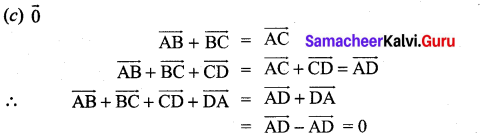Question 2.
If $$\vec{a}+2 \vec{b}$$ and $$3 \vec{a}+m \vec{b}$$ are parallel, then the value of m is ………………
(a) 3
(b) $$\frac{1}{3}$$
(c) 6
(d) $$\frac{1}{6}$$
Solution:
(c) 6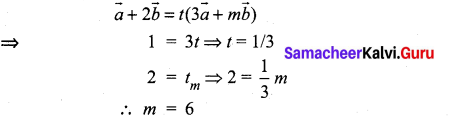Question 3.
The unit vector parallel to the resultant of the vectors $$\hat{i}+\hat{j}-\hat{k}$$ and $$\hat{i}-2 \hat{j}+\hat{k}$$ is ………………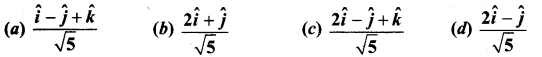Solution: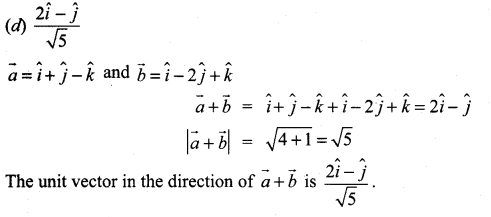Question 4.
A vector $$\overrightarrow{O P}$$ makes 60° and 45° with the positive direction of the x and y axes respectively. Then the angle between $$\overrightarrow{O P}$$ and the z-axis is …………….
(a) 45°
(b) 60°
(c) 90°
(d) 30°
Solution:
(b) 60°
α = 60°, β = 45°
We know cos2α + cos2β + cos2γ = 1
(i.e.,) $$\left(\frac{1}{2}\right)^{2}+\left(\frac{1}{\sqrt{2}}\right)^{2}$$ + cos2γ = 1
cos2γ = 1 – $$\frac{1}{4}-\frac{1}{2}=\frac{1}{4}$$
cos γ = $$\frac{1}{2}$$ ⇒ y = π/3 = 60°

Question 5.
If $$\overrightarrow{B A}=3 \hat{i}+2 \hat{j}+\hat{k}$$ and the position vector of B is $$\hat{i}+3 \hat{j}-\hat{k}$$, then the position vector A is …………………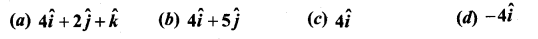Solution: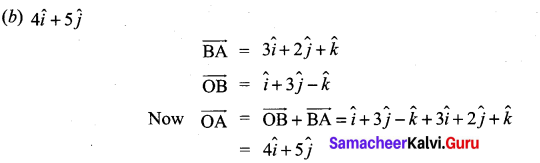Question 6.
A vector makes equal angle with the positive direction of the coordinate axes. Then each angle is equal to …………..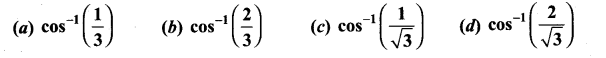Solution: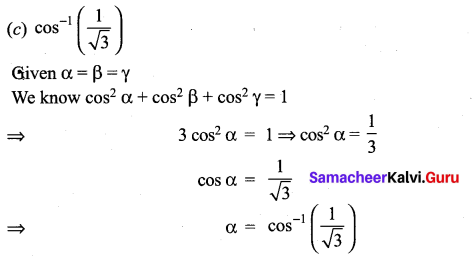Question 7.
The vectors $$\vec{a}-\vec{b}, \vec{b}-\vec{c}, \vec{c}-\vec{a}$$ are ……………
(a) parallel to each other
(b) unit vectors
(c) mutually perpendicular vectors
(d) coplanar vectors
Solution:
(d) coplanar vectors

Question 8.
If ABCD is a parallelogram, then $$\overrightarrow{A B}+\overrightarrow{A D}+\overrightarrow{C B}+\overrightarrow{C D}$$ is equal to ……………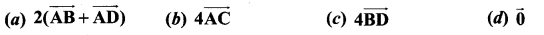Solution: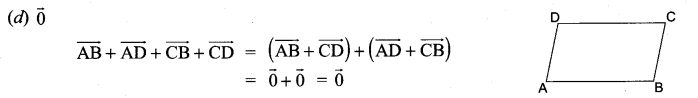Question 9.
One of the diagonals of parallelogram ABCD with $$\vec{a}$$ and $$\vec{b}$$ as adjacent sides is $$\vec{a}+\vec{b}$$. The other diagonal $$\overrightarrow{\mathrm{BD}}$$ is ……………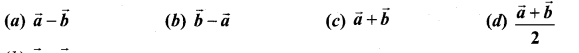Solution: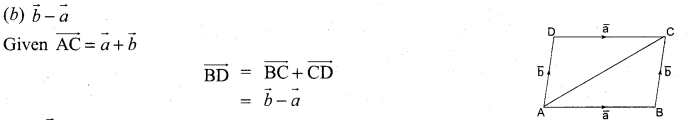Question 10.
If $$\vec{a}$$, $$\vec{b}$$ are the position vectors A and B, then which one of the following points whose position vector lies on AB, is ………….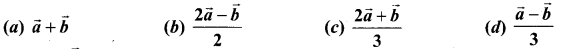Solution:
(c) $$\frac{2 \vec{a}+\vec{b}}{3}$$

Question 11.
If $$\vec{a}, \vec{b}, \vec{c}$$ are the position vectors of three collinear points, then which of the following is true?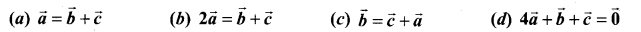Solution:
(b) $$2 \vec{a}=\vec{b}+\vec{c}$$

Question 12.
If $$\vec{r}=\frac{9 \vec{a}+7 \vec{b}}{16}$$, then the point P whose position vector $$\vec{r}$$ divides the line joining the points with position vectors $$\vec{a}$$ and $$\vec{b}$$ in the ratio ………………
(a) 7 : 9 internally
(b) 9 : 7 internally
(c) 9 : 7 externally
(d) 7 : 9 externally
Solution: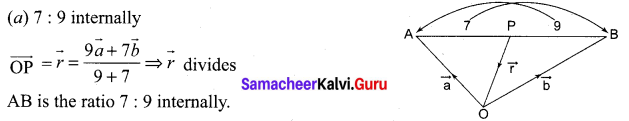Question 13.
If $$\lambda \hat{i}+2 \lambda \hat{j}+2 \lambda \hat{k}$$ is a unit vector, then the value of λ is ……………..
(a) $$\frac{1}{3}$$
(b) $$\frac{1}{4}$$
(c) $$\frac{1}{9}$$
(d) $$\frac{1}{2}$$
Solution: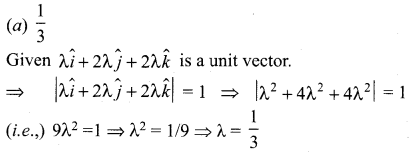Question 14.
Two vertices of a triangle have position vectors $$3 \hat{i}+4 \hat{j}-4 \hat{k}$$ and $$2 \hat{i}+3 \hat{j}+4 \hat{k}$$ .If the position vector of the centroid is $$\hat{i}+2 \hat{j}+3 \hat{k}$$, then the position vector of the third vertex is ………………….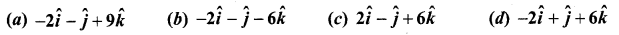Solution: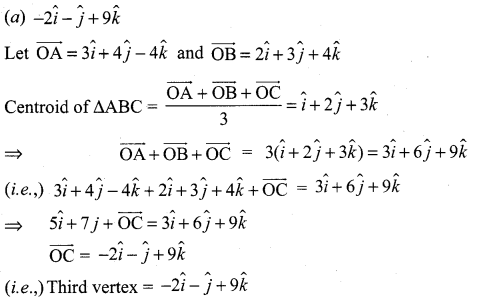Question 15.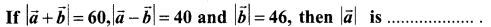(a) 42
(b) 12
(c) 22
(d) 32
Solution:
(c) 22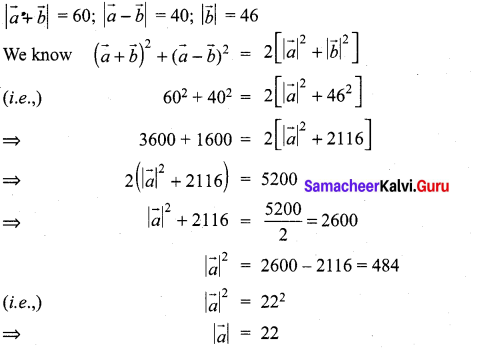Question 16.
If $$\vec{a}$$ and $$\vec{b}$$ having same magnitude and angle between them is 60° and their scalar product is $$\frac{1}{2}$$ then $$|\vec{a}|$$ is ……………
(a) 2
(b) 3
(c) 7
(d) 1
Solution:
(d) 1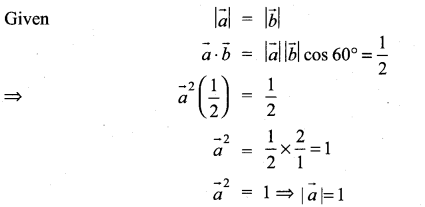Question 17.
The value of θ ∈ (0, $$\frac{\pi}{2}$$) for which the vectors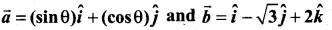are perpendicular, is equal to …………………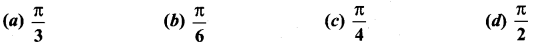Solution: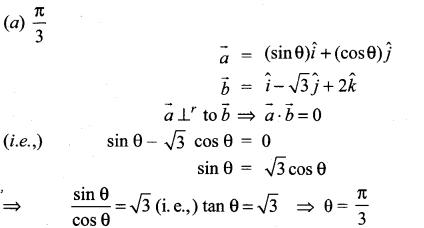Question 18.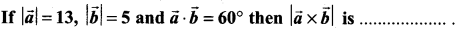(a) 15
(b) 35
(c) 45
(d) 25
Solution:
(d) 25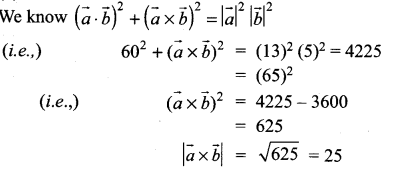Question 19.
Vectors $$\vec{a}$$ and $$\vec{b}$$ are inclined at an angle θ = 120°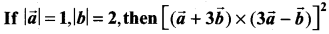is equal to ………….
(a) 225
(b) 275
(c) 325
(d) 300
Solution:
(d) 300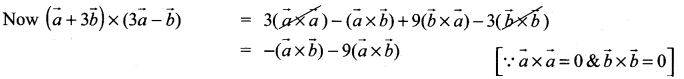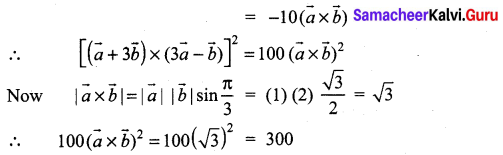Question 20.
If $$\vec{a}$$ and $$\vec{b}$$ are two vectors of magnitude 2 and inclined at an angle 60°, then the angle between $$\vec{a}$$ and $$\vec{a}+\vec{b}$$ is ………………
(a) 30°
(b)60°
(c) 45 °
(d) 90°
Solution:
(a) 30°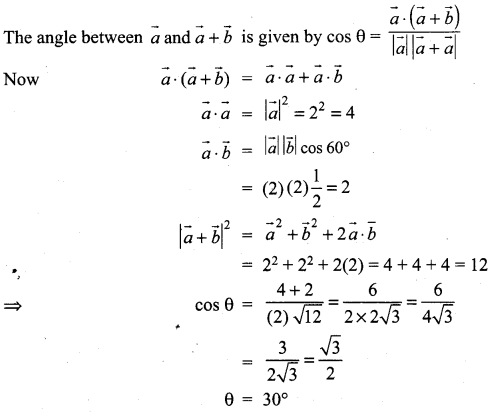Question 21.
If the projection of $$5\hat{i} -\hat{j}-3 \hat{k}$$ on the vector $$\hat{i}+3 \hat{j}+\lambda \hat{k}$$ is same as the projection of $$\hat{i}+3 \hat{j}+\lambda \hat{k}$$ on $$5\hat{i}- \hat{j}-3 \hat{k}$$ then λ is equal to ………………
(a) ±4
(b) ±3
(c) ±5
(d) ±1
Solution:
(c) ±5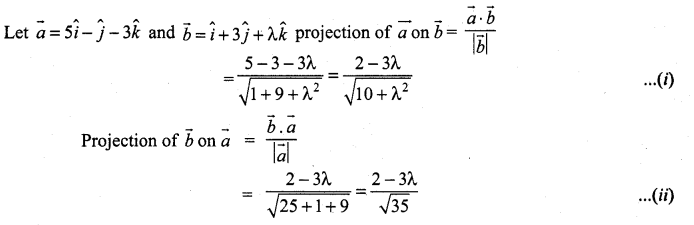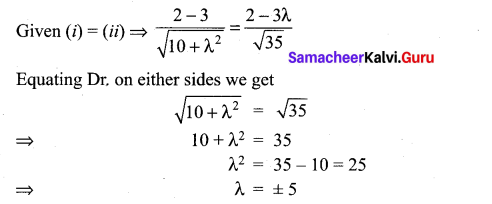Question 22.
If (1, 2, 4) and (2, – 3λ, – 3) are the initial and terminal points of the vector $$\hat{i}+5 \hat{j}-7 \hat{k}$$, then the value of λ is equal to ……………..
(a) $$\frac{7}{3}$$
(b) $$-\frac{7}{3}$$
(c) $$-\frac{5}{3}$$
(d) $$\frac{5}{3}$$
Solution: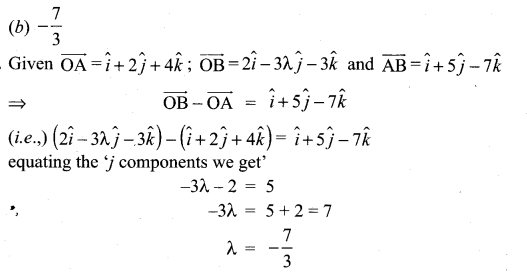Question 23.
If the points whose position vector $$10 \hat{i}+3 \hat{j}, 12 \hat{i}-5 \hat{j}$$ and $$\vec{a} \hat{i}+11 \hat{j}$$ are collinear then a is equal to ………………
(a) 6
(b) 2
(c) 5
(d) 8
Solution:
(d) 8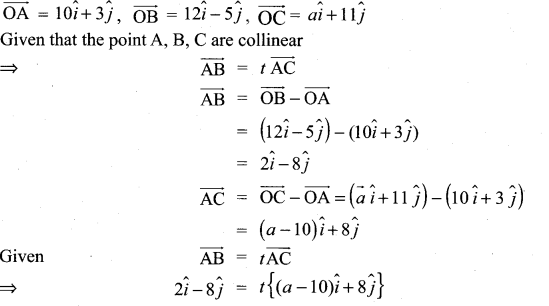equating $$\hat{j}$$ components
⇒ -8 = 8t ⇒ t = -1
equation $$\hat{i}$$ components
t(a – 10) = 2
(i.e.,) (-1) (a – 10) = 2
a – 10 = -2
a = – 2 + 10 = -8

Question 24.
If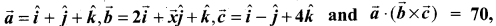then x is equal to …………..
(a) 5
(b) 7
(c) 26
(d) 10
Solution:
(c) 26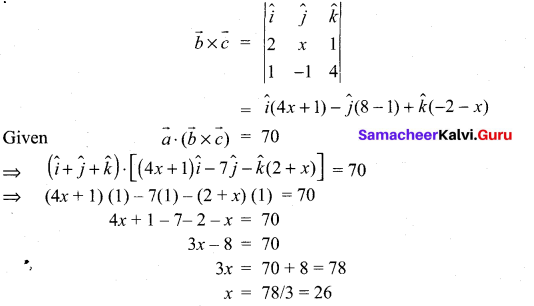Question 25.
If $$\vec{a}=\hat{i}+2 \hat{j}+2 \hat{k},|\vec{b}|=5$$ and the angle between $$\vec{a}$$ and $$\vec{b}$$ is $$\frac{\pi}{6}$$, then the area of the triangle formed by these two vectors as two sides, is …………….
(a) $$\frac{7}{4}$$
(b) $$\frac{15}{4}$$
(c) $$\frac{3}{4}$$
(d) $$\frac{17}{4}$$
Solution: